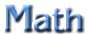Currency Converter That Quiz Funbrain Holt Online Table Trees Math Magician Times Tables Everyday Math Resources
Find Hurkle
 Interactive Math Activities Perimeter and Area IXL Perimeter Skills 2-6 Math Playground Area Perimeter Adam Ant Funbrain Area Perimeter Perimeter Explorer Shape Explorer Spy Guys (Click on Lessons) Area/Perimeter Word Problems?and Open-Ended Questions (short version) Area and Perimeter Word Problems and Open-Ended Questions (17 Qu's) Perimeter of a Rectangle AAA Math Area/Perimeter Area Explorer ? Pattern Blocks Angles Playground: Measuring Angles What's My Angle? Alien Angles Move the Mouse to Make the Angle Measuring Angles BrainPOP Angles That Quiz Time Elapsed Time Tabb Elapsed Time ? ? ? ? Every Day Math Study Links - Grade 4 Transformations Slides, Flips, Turns Mini Movies ?Key Fits BiteSize Transformations ? ? Virtual Manipulatives ? ? ? ? ? ? ? ? ? Everyday Math Resources Find Hurkle ? ? ? ? ? ? Rounding (Hangman) Funbrain Baseball Add, Subtract, Multiply, Divide ? ? Table Trees ? ? ? Order of Operations Quiz Order of Operations I (division) Basic Facts/Operations Quiz ? Math Magician times tables ? ?
 SITES WITH GRADED ACTIVITIES OR VARIETIES OF ACTIVITES COVERING MANY GRADE LEVELS AAA MATH ? COOL MATH 4 KIDS A+ MATH VIRTUAL MANIPULATIVES IXL MATH PRACTICE (Pre-K - 3) LEARNING PLANET Math Games by Grade Level MATH PLAYGROUND MANIPULATIVES MATH PLAYGROUND WORD PROBLEMS SIMON TEACH THE CHILDREN WELL MATH ? ? ? ?

?

 GRADES 1 AND 2 GRADES 3 AND 4 GRADES 5 AND 6 Make Your Own Fraction Identify Fractions on a Circle Table Trees Order of Operations I (division) Spy Guys Lessons Area and Perimeter Word Problems and Open Ended Qu's Paintbrush Math Lines of Symmetry Math Magician times tables Basic Facts/Operations Quiz Order of Operations Quiz Comparing Fractions and Decimals Basic Facts/Operations Quiz Math Dojo (Select "Maybe Later" for free activity) Elapsed Time Visual Fractions Angles and Protractors Instruction and Practice ? ? Visual Fractions Math Playground? Find Hurkle Elapsed Time ?

?